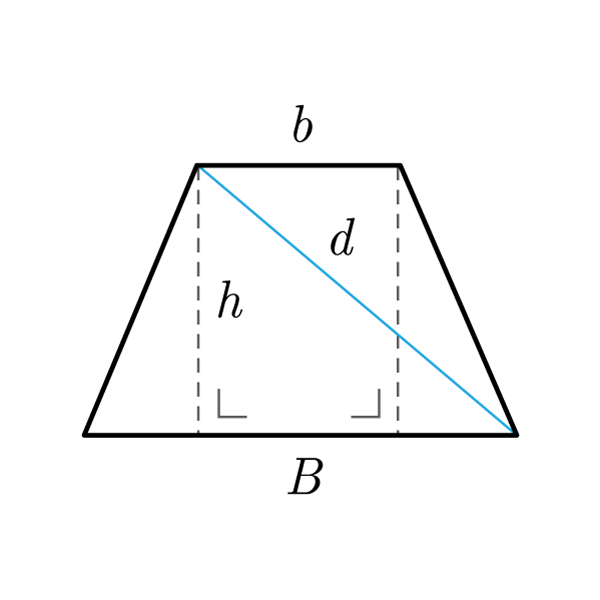# Isosceles Trapezoid

 Longer Base $$B$$ Shorter Base $$b$$ Height $$h$$ Oblique Side $$S$$ Oblique side Projection $$p_{1}$$ Diagonal $$d$$
$$2p = B + b + 2S$$
Perimeter
$$A = \frac{\left(B + b \right) \times h}{2}$$
Area
$$B + b = \frac{2A}{h}$$
Sum of bases
$$h = \frac{2A}{B + b}$$
Height
$$B + b = 2p - 2S$$
Sum of bases
$$S = \frac{2p - B - b}{2}$$
Oblique Side
$$p_{1} = \frac{ B - b }{2}$$
Oblique side Projection
$$B - b = 2 \times p_{1}$$
Difference of bases
$$B = b + 2p_{1}$$
Longer Base
$$b = B - 2p_{1}$$
Shorter Base
Right Tr. delimited by height - oblique side
$$S = \sqrt{ {p_{1}}^2 + {h}^2 }$$
Side (Pythagoras' theorem)
$$h = \sqrt{ {S}^2 - {p_{1}}^2 }$$
Height
$$p_{1} = \sqrt{ {S}^2 - {h}^2 }$$
Oblique side Projection

## Definition

An isosceles trapezoid is a trapezoid with oblique sides congruent.

## Properties

1. The oblique sides are congruent
2. The angles adjacent to their respective bases are congruent
3. Diagonals are congruent
4. All the  Generic Trapezoid formulas are valid## Isosceles Trapezoid Formulas

Data Formula
Perimeter 2p = B + b + 2 × S
Area A = [(B + b) × h] / 2
Height h = (2 × A) / (B + b)
Oblique Side S = (2p - B - b) / 2
Oblique side Projection p1 = (B - b) / 2
Sum of bases B + b = (2 × A) / h
Sum of bases B + b = 2p - 2 × S